$$\require{cancel}$$

# 13.4: Chapter 4

Problem (4.1)

A current I amps flows in the inner conductor of an infinitely long co-axial line and returns via the outer conductor. The radius of the inner conductor is a, and b and c are the inner and outer radii of the outer conductor (see the sketch). The current density is uniform in the two conductors. Calculate the magnetic flux density in all regions. The magnetization density can be set equal to zero everywhere.This problem exhibits cylindrical symmetry so that it is ideal for an application of Stokes' theorem. Let z be the direction along the cable. Then there is only a component Az of the vector potential $$\left(\mathbf{A}=\frac{\mu_{0} I}{4 \pi} \oint_{C} \frac{d \mathbf{L}}{r}\right)$$. Moreover, by symmetry Az cannot depend upon the angle θ, nor can it depend upon z (infinite wire).

∴ Az = Az(r).

In cylindrical co-ordinates

$$\operatorname{curl} \mathbf{A}=\frac{1}{r}\left|\begin{array}{ccc} \hat{\mathbf{u}}_{r} & r \hat{\mathbf{u}}_{\theta} & \hat{\mathbf{u}}_{z} \\ \frac{\partial}{\partial r} & \frac{\partial}{\partial \theta} & \frac{\partial}{\partial z} \\ A_{r} & r A_{\theta} & A_{z} \end{array}\right|$$ , ∴ B has only a θ component

$$\text{B}_{\theta}=-\frac{\partial \text{A}_{z}}{\partial \text{r}}$$.

But since there is no magnetization and no time dependence

Curl B = µo Jf

$$\therefore \int_{\text {surface }} \operatorname{curl} \mathbf{B} \cdot \mathbf{d s}=\mu_{0} \int_{\text {surface }} \mathbf{J}_{f}\cdot \mathbf{d s}$$

or

$$\oint_{C} \mathbf{B} \cdot d \mathbf{L}=\mu_{0} \int_{\text {surface }} \mathbf{J}_{f} \cdot \mathbf{d s}$$

Apply this to a circle of radius r:

Case (1) $$r \leq a \quad J_{f}=\frac{I}{\pi a^{2}}$$

$$\therefore 2 \pi r B_{\theta}=\mu_{0}\left(\frac{I}{\pi a^{2}}\right)\left(\pi r^{2}\right)=\mu_{0} I\left(\frac{r}{a}\right)^{2}$$

$$\therefore \quad B_{\theta}=\left(\frac{\mu_{0} I}{2 \pi a^{2}}\right) r$$

So when r = 0 Bθ = 0

when r = a $$\text{B}_{\theta}=\frac{\mu_{0} I}{2 \pi a}$$.

Case (2) a ≤ r ≤ b

In this case $$\int_{\text {surface }} \mathbf{J}_{f} \cdot \mathbf{ds} \equiv \text{I}$$

∴ 2$$\pi$$r Bθ = µo I

$$\text{B}_{\theta}=\frac{\mu_{0} I}{2 \pi r}$$

When r=a $$B_{\theta}=\frac{\mu_{0} I}{2 \pi a}$$

When r=b $$B_{\theta}=\frac{\mu_{0} I}{2 \pi b}$$

Case (3) b ≤ R ≤ c

In the outer conductor

$$\left|J_{f}\right|=\frac{I}{\pi\left(c^{2}-b^{2}\right)}$$

and the current flow is negative. Therefore this time one has

$$2 \pi r B_{\theta}=\mu_{0}\left[I-\frac{I \pi\left(r^{2}-b^{2}\right)}{\pi\left(c^{2}-b^{2}\right)}\right]$$

$$B_{\theta}=\frac{\mu_{0} I}{2 \pi r}\left[1-\left(\frac{r^{2}-b^{2}}{c^{2}-b^{2}}\right)\right]$$

So when r = b $$\text{B}_{\theta}=\frac{\mu_{0} I}{2 \pi \text{b}}$$

When r = c Bθ = 0

Case (4) R ≥ C

Here 2$$\pi$$rBθ = µo(I - I) ≡ 0 ∴ Bθ = 0.

There is no field outside this co-axial cable. Notice that the tangential component of B is continuous across the boundaries.

Problem (4.2)

Two identical coaxial coils, each of N turns and radius a, are separated by a distance d as shown in the sketch. A current flows through each coil so that the fields of the two coils add at the origin.

(a) Calculate Bz at the origin

(b) Show that $$\frac{d B z}{d z}=0$$ at z = 0.

(c) Find d such that $$\frac{d^{2} B_{z}}{d z^{2}}=0$$ at z = 0.

Such a configuration is the simplest system for generating a uniform magnetic field. It is known as a Helmholtz pair.The field of a single coil along its axis is

$B_{z}=\frac{\mu_{0} N I}{2} \frac{a^{2}}{\left(a^{2}+z^{2}\right)^{3 / 2}}\nonumber$

where z is measured from the center of the coil. For the above pair of coils

$\text{B}_{\text{z}}=\frac{\mu_{\text{o}} \text{NIa}^{2}}{2}\left\{\frac{1}{\left[\left(z-\frac{\text{d}}{2}\right)^{2}+\text{a}^{2}\right]^{3 / 2}}+\frac{1}{\left[\left(z+\frac{\text{d}}{2}\right)^{2}+\text{a}^{2}\right]^{3 / 2}}\right\}\nonumber$

(a) At z = 0 $$\text{B}_{\text{z}}=\mu_{\text{0}} \text{NI} \left(\frac{\text{a}^{2}}{\left[\frac{\text{d}^{2}}{4}+\text{a}^{2}\right] 3 / 2}\right)$$

(b) $\frac{d B_{z}}{d z} \propto \frac{-3\left(z-\frac{d}{2}\right)}{\left[\left(z-\frac{d}{2}\right)^{2}+a^{2}\right] 5 / 2}-\frac{3\left(z+\frac{d}{2}\right)}{\left[\left(z+\frac{d}{2}\right)^{2}+a^{2}\right] 5 / 2}\nonumber$

Thus at z = 0 $$\frac{d B_{z}}{d z}=0$$.

(c) $\frac{d^{2} B_{z}}{d z^{2}} \propto \frac{-3}{\left[\left(z-\frac{d}{2}\right)^{2}+a^{2}\right]^{5 / 2}}-\frac{3}{\left[\left(z+\frac{d}{2}\right)^{2}+a^{2}\right] 5 / 2}+\frac{15\left(z-\frac{d}{2}\right)^{2}}{\left[\left(z-\frac{d}{2}\right)^{2}+a^{2}\right]^{7 / 2}}+\frac{15\left(z+\frac{d}{2}\right)^{2}}{\left[\left(z+\frac{d}{2}\right)^{2}+a^{2}\right]^{7 / 2}}\nonumber$

∴ at z = 0

$\begin{array}{c} \frac{d^{2} B_{z}}{d z^{2}} \propto \frac{-3\left(\frac{d^{2}}{4}+a^{2}\right)-3\left(\frac{d^{2}}{4}+a^{2}\right)+15\left(\frac{d^{2}}{4}\right)+15\left(\frac{d^{2}}{4}\right)}{\left[\frac{d^{2}}{4}+a^{2}\right]^{7 / 2}} \\ \propto 6 d^{2}-6 a^{2}=6\left(d^{2}-a^{2}\right) \end{array}\nonumber$

So $$\frac{d^{2} B_{z}}{d z^{2}}=0$$ if d = a.

Thus for a Helmholtz pair d = a.

The magnetic field strength at the center of the Helmholtz pair is given by

$$B_{z}(0)=\frac{\mu_{0} N I}{a} \left(\frac{4}{5}\right)^{3 / 2}=0.716 \frac{\mu_{0} N I}{a}$$.

Problem (4.3)

A solenoid is 1 meter long and it carries 104 turns of wire. The average radius of the coil is 0.1 meters. The coil carries a current of 10 Ampères.

(a) Calculate the field at the center of the solenoid.

(b) If the wire of the coil has a cross-sectional area of 10-6 meters2 calculate the resistance of the coil. R = ρL/A and for copper ρ = 2 x 10-8 ohm meters.

(c) How much power is required to produce the magnetic field of part (a)?

This calculation explains why iron core magnets are used to generate fields of ~ 1 Tesla.

$\text{B}_{\text{z}}(\text{z})=\frac{\mu_{\text{o}} \text{NI}}{2}\left\{\frac{(\text{z}+\text{L} / 2)}{\sqrt{\text{R}^{2}+\left(\text{z}+\frac{\text{L}}{2}\right)^{2}}}-\frac{(\text{z}-\text{L} / 2)}{\sqrt{\text{R}^{2}+\left(\text{z}-\frac{\text{L}}{2}\right)^{2}}}\right\}\nonumber$

N is the number of turns/m, L the length of the coil.

At z = 0 $$\text{B}_{\text{z}}(\text{o})=\frac{\mu_{\text{o}} \text{NI}}{2} \frac{\text{L}}{\sqrt{\text{R}^{2}+(\text{L} / 2)^{2}}}$$

Here N = 104/m, I = 10 Amps, $$\frac{L}{2}=\frac{1}{2} m$$, and R = 0.1 m

(a) $$\therefore B_{z}(0)=\frac{\left(2 \pi \times 10^{-2}\right)}{\sqrt{.01+.25}}=0.123 \text { Tesla }$$ i.e. ~ 103 x earth's field!

(b) L = (2$$\pi$$R)(104) = 6.283 x 103 m ∴ R = 125.7 Ohms

(c) For 10 Amps one would require 1257 Volts and a power = VI = 12,570 Watts!! = 12.57 kWatts!

Problem (4.4)

A square loop of wire 1 cm on a side carries a current of 2 Ampères.

(a) Estimate the magnitude of the magnetic field on the axis of the current loop and 1 meter from its center. The loop may be treated like a point dipole.

(b) Estimate the magnitude and direction of the magnetic field one meter from the center of the loop but at a point in the plane of the loop.

The magnetic moment of the loop is Mo = IA = (2)(10-4) Amp m2.

Now $$\mathbf{B}=\frac{\mu_{0}}{4 \pi}\left[\frac{3(\mathbf{m} \cdot \mathbf{r}) \mathbf{r}}{r^{5}}-\frac{\mathbf{m}}{r^{3}}\right]$$

(a) On the axis of the dipole m$$\cdot$$r = Mor

So $$B_{z}=\left(\frac{\mu_{0}}{4 \pi}\right) \left(\frac{2 M_{0}}{r^{3}}\right)=4 \times 10^{-11} \text {Tesla }$$

(The earth's field is ~ 10-4 Tesla so this is very weak).

(b) On the equatorial plane m$$\cdot$$r = 0

$$\therefore B_{z}=-\frac{\mu_{0}}{4 \pi} \frac{M_{0}}{r^{3}}=-2 \times 10^{-11} \text {Tesla }$$

Directed opposite to the dipole moment.

Problem (4.5).

Calculate the magnetic field along the z-axis of a square coil which carries a current of I Amps (see the sketch).Each side of the square is 2L meters long.

Along the axis of the coil there will be only a zcomponent of magnetic field by symmetry. In order to get the total field it is only necessary to calculate the z-component of the field generated by one side of the coil and then multiply by four. Consider the right hand side.

Let $$d \mathbf{L}=d y \hat{\mathbf{u}}_{y} \quad \text { at } \quad(L, Y)$$

The position of the element of length, dL, is specified by r where $$\mathbf{r}=\text{L} \hat{\mathbf{u}}_{\text{x}}+\text{y} \hat{\mathbf{u}}_{\text{y}}$$. The position of the point of observation along the z-axis is specified by $$\mathbf{R}=z \hat{\mathbf{u}}_{z}$$.

Therefore,

$$(\mathbf{R}-\mathbf{r})=-L \hat{\mathbf{u}}_{\text{x}}-\text{y} \hat{\mathbf{u}}_{\text{y}}+\text{z} \hat{\mathbf{u}}_{\text{z}}$$

and

$$|\mathbf{R}-\mathbf{r}|=\sqrt{L^{2}+y^{2}+z^{2}}$$.

From the law of Biot-Savard one obtains

$d\mathbf{B}=\frac{\mu_{0}}{4 \pi} I \frac{\text{d} \mathbf{L} \times(\mathbf{R}-\mathbf{r})}{|\mathbf{R}-\mathbf{r}|^{3}}\nonumber;$

from which

$\text{d} \text{B}_{\text{z}}=\frac{\mu_{0}}{4 \pi} \text{I} \frac{\text{L} \text{d} \text{y}}{\left(\text{L}^{2}+\text{y}^{2}+\text{z}^{2}\right)^{3 / 2}}\nonumber,$

and

$B_{z}=\frac{\mu_{0}}{4 \pi} I \frac{2 L^{2}}{\left(z^{2}+L^{2}\right) \sqrt{z^{2}+2 L^{2}}}\nonumber.$

This must be multiplied by 4x because the coil has four sides:

$\text{B}_{\text{z}}(\text{z})=\frac{2 \mu_{0} \text{I}}{\pi} \frac{\text{L}^{2}}{\left(\text{z}^{2}+\text{L}^{2}\right) \sqrt{\text{z}^{2}+2 \text{L}^{2}}}\nonumber.$

At z=0 $$\text{B}_{\text{z}}(0)=\frac{2 \sqrt{2}}{\pi} \frac{\mu_{0} I}{2 \text{L}}=0.9003 \frac{\mu_{0} I}{2 \text{L}}$$

This value can be compared with $$B_{z}(0)=\frac{\mu_{0} I}{2 R}$$ for a circular coil.

Problem (4.6).

(a) How far apart should two square coils be mounted in order to obtain as homogeneous as possible a magnetic field? See the sketch.( One wants $$\frac{d^{2} B_{z}}{d z^{2}}=0$$ at the center of the coil system. With a little thought one can convince oneself that at z=0 the quantity $$\frac{d^{2} B_{z}}{d z^{2}}$$ is exactly the same for each coil, so that the work of differentiation can be reduced by a factor two.)

(b) Over what distance along the z-axis will the field deviate by less than 1% from the field at the center of the coil system if L= 1 meter?

Such square coils are often more convenient to build than circular coils if the earth's magnetic field is to be cancelled over a large volume.

(a) From the results of Problem (3.5) one can write

$$\mathrm{B}_{\mathrm{z}}(\mathrm{z})=\frac{2 \mu_{0} \mathrm{I\ L^2}}{\pi}\left(\Psi_{1}+\Psi_{2}\right)$$, where

$$\Psi_{1}=\frac{1}{\left((z+d)^{2}+L^{2}\right)\left((z+d)^{2}+2 L^{2}\right)^{1 / 2}}$$,

and $$\psi_2=\frac{1}{\left((z-d)^{2}+L^{2}\right)\left((z-d)^{2}+2 L^{2}\right)^{1 / 2}}$$.

$$\frac{d \psi_{1}}{d z}=\frac{-(z+d)\left(3(z+d)^{2}+5 L^{2}\right)}{\left((z+d)^{2}+L^{2}\right)^{2}\left((z+d)^{2}+2 L^{2}\right)^{3 / 2}}$$, and

$$\frac{d \Psi_{2}}{d z}=\frac{-(z-d)\left(3(z-d)^{2}+5 L^{2}\right)}{\left((z-d)^{2}+L^{2}\right)^{2}\left((z-d)^{2}+2 L^{2}\right)^{3 / 2}}$$.

Note that at z=0 $$\frac{d}{d z}\left(\psi_{1}+\psi_{2}\right) \equiv 0$$; the field gradient vanishes by symmetry.

$$\frac{d^{2} \Psi_{1}}{d z^{2}}=\frac{N}{D}$$, where

\begin{aligned} N=-&\left(9(z+d) 2+5 L^{2}\right)\left((z+d)^{2}+L^{2}\right)\left((z+d)^{2}+2 L^{2}\right) \\ &+\left(12(z+d)^{4}+20 L^{2}(z+d)^{2}\right)\left((z+d)^{2}+2 L^{2}\right) \\ &+\left(9(z+d)^{4}+15 L^{2}(z+d)^{2}\right)\left((z+d)^{2}+L^{2}\right) \end{aligned}\nonumber

and

$D=\left((z+d)^{2}+L^{2}\right)^{3}\left((z+d)^{2}+2 L^{2}\right)^{5 / 2}\nonumber.$

At $$z=0 \frac{d^{2} \psi_{1}}{d z^{2}}=\frac{d^{2} \psi_{2}}{d z^{2}}$$ so that for optimum uniformity We require the numerator in the second derivative to vanish at z=0. This condition gives

$$\eta^{6}+3 \eta^{4}+\left(\frac{11}{6}\right) \eta^{2}-\left(\frac{5}{6}\right)=0$$ (1)

where $$\eta=(d / L)$$. The solution is $$\frac{d}{L}=0.5445057$$ (see the figure below). The coils should be placed 2d= 1.0890L apart.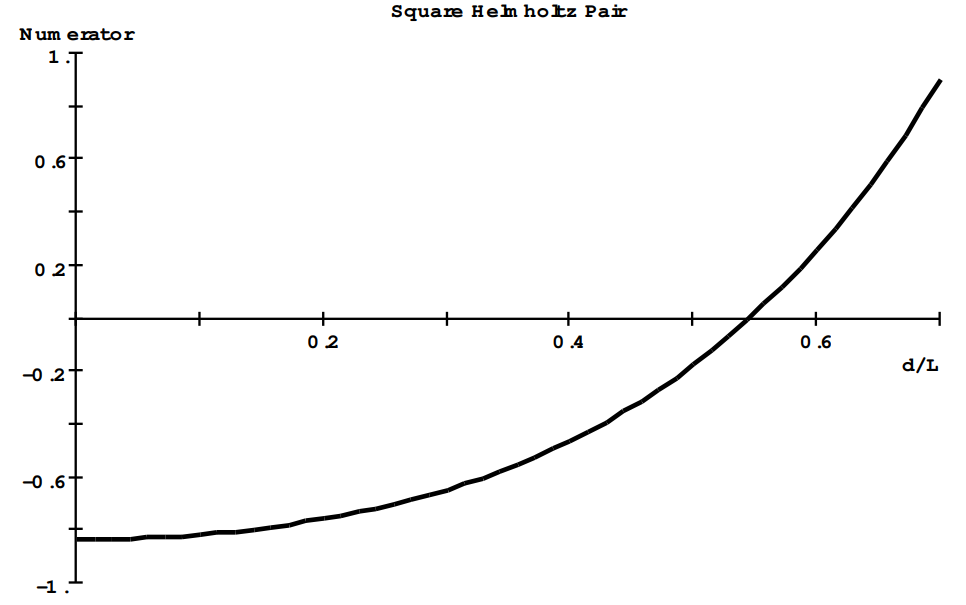(b) The simplest way to examine the homogeneity is to plot the field function:

$\text{B}_{z}(\zeta)=\frac{2 \mu_{0} I}{\pi \text{L}}\left(\frac{1}{\left((\zeta+\eta)^{2}+1\right) \sqrt{(\zeta+\eta)^{2}+2}}+\frac{1}{\left((\zeta-\eta)^{2}+1\right) \sqrt{(\zeta-\eta)^{2}+2}}\right)\nonumber,$

where ζ= (z/L) and where $$\eta$$= (d/L)= 0.5445057. At the center of the coil system

$$\text{B}_{\text{z}}(0)=\frac{2 \mu_{0} I}{\pi \text{L}}(1.017958) \text { Teslas. }$$From this graph one finds that the field has decreased by 1% when $$\left(\frac{z}{L}\right)=0.344$$. This means that the field varies by less than 1% over a central region whose length is 0.688L. It turns out that the field is homogeneous to within 1% within a volume whose diameter is 0.688L: i.e. within the sphere whose diameter is ~68 cm if L=1 meter.

Problem (4.7)

Consider a square loop of wire lying in the xy-plane as shown in the sketch. The loop carries a current of I amps and is centered on the origin.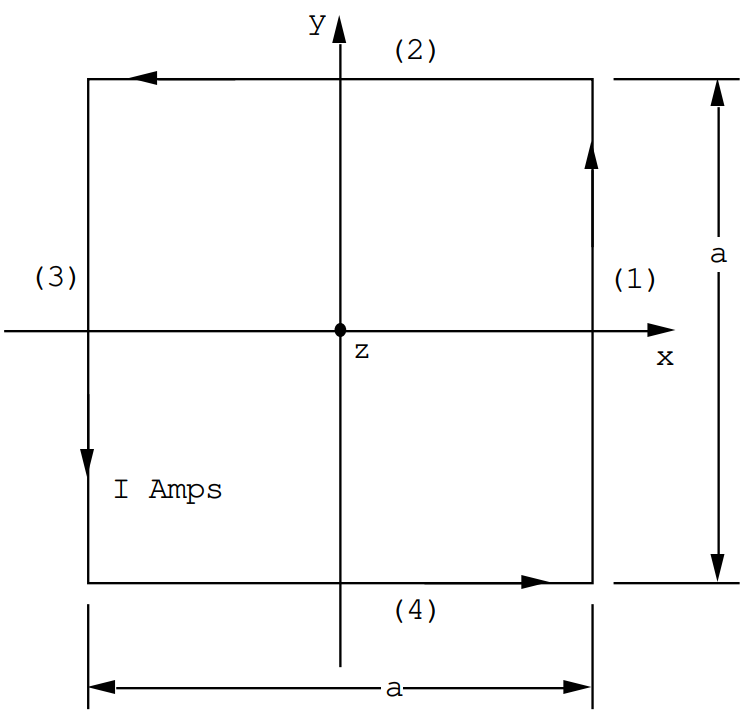(a) Show that the contribution to the vector potential at a point P(X,Y,Z) from side (1) has only a y component and that this component is given by

$\text{A}_{\text{Y} 1}=\frac{\mu_{0} I}{4 \pi} \ln \left(\frac{\text{Y}-(\text{a} / 2)-\sqrt{(\text{X}-\text{a} / 2)^{2}+(\text{Y}-\text{a} / 2)^{2}+\text{Z}^{2}}}{\text{Y}+(\text{a} / 2)-\sqrt{(\text{X}-\text{a} / 2)^{2}+(\text{Y}+\text{a} / 2)^{2}+\text{Z}^{2}}}\right)\nonumber.$

(b) Show that the contribution to the vector potential at a point P(X,Y,Z) from side (3) has only a y component and that this component is given by

$\text{A}_{\text{Y} 3}=-\frac{\mu_{0} \text{I}}{4 \pi} \ln \left(\frac{\text{Y}-(\text{a} / 2)-\sqrt{(\text{X}+\text{a} / 2)^{2}+(\text{Y}-\text{a} / 2)^{2}+\text{Z}^{2}}}{\text{Y}+(\text{a} / 2)-\sqrt{(\text{X}+\text{a} / 2)^{2}+(\text{Y}+\text{a} / 2)^{2}+\text{Z}^{2}}}\right)\nonumber.$

(c) Show that the contribution to the vector potential at a point P(X,Y,Z) from side (2) has only an x component and that this component is given by

$\text{A}_{\text{X} 2}=-\frac{\mu_{0} I}{4 \pi} \ln \left(\frac{\text{X}-(\text{a} / 2)-\sqrt{(\text{X}-\text{a} / 2)^{2}+(\text{Y}-\text{a} / 2)^{2}+\text{Z}^{2}}}{\text{X}+(\text{a} / 2)-\sqrt{(\text{X}+\text{a} / 2)^{2}+(\text{Y}-\text{a} / 2)^{2}+\text{Z}^{2}}}\right)\nonumber.$

(d) Show that the contribution to the vector potential at a point P(X,Y,Z) from side (4) has only an x component and that this component is given by

$\text{A}_{\text{X} 4}=\frac{\mu_{0} I}{4 \pi} \ln \left(\frac{\text{X}-(\text{a} / 2)-\sqrt{(\text{X}-\text{a} / 2)^{2}+(\text{Y}+\text{a} / 2)^{2}+\text{Z}^{2}}}{\text{X}+(\text{a} / 2)-\sqrt{(\text{X}+\text{a} / 2)^{2}+(\text{Y}+\text{a} / 2)^{2}+\text{Z}^{2}}}\right)\nonumber.$

(e) Now consider the point P(X,0,Z) which is specified by the vector $$\mathbf{R}=\text{X} \hat{\mathbf{u}}_{\mathbf{X}}+\text{Z} \hat{\mathbf{u}}_{\mathbf{z}}$$. Show that

Ax = 0,

$\text{A}_{\text{y}}=\frac{\mu_{0} \text{I}}{4 \pi} \ln ((\frac{1+\frac{a}{2 \sqrt{(x-a / 2)^{2}+\frac{a^{2}}{4}+z^{2}}}}{1-\frac{a}{2 \sqrt{(X-a / 2)^{2}+\frac{a^{2}}{4}+Z^{2}}}})(\frac{1-\frac{a}{2 \sqrt{(x+a / 2)^{2}+\frac{a^{2}}{4}+z^{2}}}}{1+\frac{a}{2 \sqrt{(x+a / 2)^{2}+\frac{a^{2}}{4}+z^{2}}}}))\nonumber.$

In the limit as a/R→0, where $$\text{R}=\sqrt{\text{X}^{2}+\text{Z}^{2}}$$, the expression for Ay can be shown to go to the limit

$\text{A}_{\text{y}}=\frac{\mu_{0} \operatorname{Ia}^{2}}{4 \pi}\left(\frac{\text{X}}{\text{R}^{3}}\right)=\frac{\mu_{0}}{4 \pi}\left(\frac{\text{m}_{\text{z}} \text{x}}{\text{R}^{3}}\right)\nonumber,$

where mz= Ia2 Amp-meters2. This is just the x-component of the expression $$\mathbf{A}=\frac{\mu_{0}}{4 \pi} \frac{\mathbf{m} \times \mathbf{R}}{R^{3}}$$, the dipole vector potential.

We shall show the calculation for side (1). The procedure for the other three sides is very similar. For side (1) the element of length is given by

$$\text{d} \mathbf{L}=\text{d} \text{y} \hat{\mathbf{u}}_{\text{y}}$$.

This element is located at $$\mathbf{r}=\frac{a}{2} \hat{\mathbf{u}}_{x}+y \hat{\mathbf{u}}_{y}$$. The point of observation is located at $$\mathbf{R}=\text{X} \hat{\mathbf{u}}_{\text{x}}+\text{Y} \hat{\mathbf{u}}_{\text{y}}+\text{z} \hat{\mathbf{u}}_{\text{z}}$$, therefore

$\mathbf{R}-\mathbf{r}=\left(\text{X}-\frac{\text{a}}{2}\right) \hat{\mathbf{u}}_{\text{x}}+(\text{Y}-\text{y}) \hat{\mathbf{u}}_{\text{y}}+\text{Z} \hat{\mathbf{u}}_{\text{z}}\nonumber.$

The length of this line is given by

$|\mathbf{R}-\mathbf{r}|=\sqrt{\left(X-\frac{a}{2}\right)^{2}+(Y-Y)^{2}+Z^{2}}\nonumber.$

The contribution to the vector potential at P has only a y-component because the current element has only a y component:

$\text{d} \text{A}_{\text{y}}=\frac{\mu_{0} \text{I}}{4 \pi} \frac{\text{d} \text{y}}{\sqrt{\left(\text{X}-\frac{\text{a}}{2}\right)^{2}+(\text{Y}-\text{y})^{2}+\text{Z}^{2}}}, \quad \text { and }\nonumber$

$\text{A}_{\text{y}}=\frac{\mu_{0} I}{4 \pi} \int_{-\text{a} / 2}^{\text{a} / 2} \frac{\text{d} \text{y}}{\sqrt{\left(\text{X}-\frac{\text{a}}{2}\right)^{2}+(\text{Y}-\text{y})^{2}+\text{Z}^{2}}}\nonumber.$

This is a standard integral; it can be written

$\text{A}_{\text{Y} 1}=\frac{\mu_{0} I}{4 \pi} \ln \left(\frac{\text{Y}-(\text{a} / 2)-\sqrt{(\text{X}-\text{a} / 2)^{2}+(\text{Y}-\text{a} / 2)^{2}+\text{Z}^{2}}}{\text{Y}+(\text{a} / 2)-\sqrt{(\text{X}-\text{a} / 2)^{2}+(\text{Y}+\text{a} / 2)^{2}+\text{Z}^{2}}}\right)\nonumber.$

(e) The expansion for Ay in the limit of (a/R)→0 can be carried out as follows: ( it is convenient to use the notation

$\sqrt{(x-a / 2)^{2}+\frac{a^{2}}{4}+z^{2}}=\sqrt{x^{2}+z^{2}-a x+\frac{a^{2}}{2}}=R_{-}\nonumber,$

and

$\sqrt{(x+a / 2)^{2}+\frac{a^{2}}{4}+z^{2}}=\sqrt{x^{2}+z^{2}+a x+\frac{a^{2}}{2}}=R_{+}\nonumber.$

$\ln \left(\frac{\left(1+\frac{a}{2 R_{-}}\right)\left(1-\frac{a}{2 R_{+}}\right)}{\left(1-\frac{a}{2 R_{-}}\right)\left(1+\frac{a}{2 R_{+}}\right)}\right)=\ln \left(1+\frac{a}{2 R_{-}}\right)+\ln \left(1-\frac{a}{2 R_{+}}\right)-\ln \left(1-\frac{a}{2 R_{-}}\right)-\ln \left(1+\frac{a}{2 R_{+}}\right)\nonumber.$

Expand to first order in small quantities:

$$\cong \frac{a}{R_{-}}-\frac{a}{R_{+}}$$,

since (a/R-) and (a/R+) are very small. One can finally write

$\left(a / R_{-}\right)=\frac{a}{R}\left(1-\frac{a X}{R^{2}}+\frac{a^{2}}{2 R^{2}}\right)^{-1 / 2} \cong \frac{a}{R}\left(1+\frac{a X}{2 R^{2}}-\frac{a^{2}}{4 R^{2}}\right)\nonumber,$

$\left(a / R_{+}\right)=\frac{a}{R}\left(1+\frac{a X}{R^{2}}+\frac{a^{2}}{2 R^{2}}\right)^{-1 / 2} \cong \frac{a}{R}\left(1-\frac{a X}{2 R^{2}}-\frac{a^{2}}{4 R^{2}}\right)\nonumber,$

where $$\text{R}=\sqrt{\text{X}^{2}+\text{Z}^{2}}$$.

It follows from this that to first order in small quantities

$\text{A}_{\text{Y}}=\frac{\mu_{0} I}{4 \pi} \frac{\text{a}^{2} \text{X}}{\text{R}^{3}}\nonumber.$

Problem (4.8)

A short cylindrical solenoid has a radius of R= 5x10-2 meters and a length of L= 5x10-2 meters. It is wound with N= 8x104 turns/meter, and the windings carry a current of I= 10 Amps.

(a) What is the magnetic field at the center of the solenoid?

(b) What is the magnetic field strength on the axis of the solenoid but at the end face (z=L/2)?

The magnetic field along the axis of a short solenoid is given by (z is measured from the solenoid center)

$\text{B}_{\text{z}}(\text{z})=\frac{\mu_{0} \text{NI}}{2}\left(\frac{(\text{z}+\text{L} / 2)}{\sqrt{(\text{z}+\text{L} / 2)^{2}+\text{R}^{2}}}+\frac{(\text{L} / 2-\text{z})}{\sqrt{(\text{z}-\text{L} / 2)^{2}+\text{R}^{2}}}\right)\nonumber.$

(a) At z=0 $$B_{z}(0)=\frac{\mu_{0} N I}{2} \frac{L}{\sqrt{(L / 2)^{2}+R^{2}}}$$

For this problem $$\frac{\mu_{0} \text{NI}}{2}=0.503 \text { Teslas. }$$.

Therefore Bz(0)= 0.450 Teslas.

(b) At z= L/2= 2.5x10-2 meters:

$\text{B}_{\text{z}}(\text{L} / 2)=\frac{\mu_{0} \text{NI}}{2}\left(\frac{\text{L}}{\sqrt{\text{L}^{2}+\text{R}^{2}}}\right)=0.707\left(\frac{\mu_{0} \text{NI}}{2}\right)=0.356 \text { Teslas }\nonumber.$

Problem (4.9)

A short cylindrical disc has a radius of R= 5x10-2 meters and a length of L= 5x10-2 meters. It is uniformly magnetized; the magnetization density is parallel with the axis of the disc, the z-axis, and the magnetization has the value M0= 0.955x106 Amps/meter.

(a) What is the magnetic field at the center of the disc?

(b) What is the magnetic field strength on the axis of the disc but at the end face (z=L/2)?

The magnetic field distribution generated by a uniformly magnetized disc is the same as that generated by the windings of a short solenoid. The magnetic field along the axis of a short solenoid is given by

$\text{B}_{\text{z}}(\text{z})=\frac{\mu_{0} \text{NI}}{2}\left(\frac{(\text{z}+\text{L} / 2)}{\sqrt{(\text{z}+\text{L} / 2)^{2}+\text{R}^{2}}}+\frac{(\text{L} / 2-\text{z})}{\sqrt{(\text{z}-\text{L} / 2)^{2}+\text{R}^{2}}}\right)\nonumber.$

It is only necessary to replace the product NI in this formula by the magnetization M0.

(a) At z=0 $$\text{B}_{\text{z}}(0)=\frac{\mu_{0} \text{M}_{0}}{2} \frac{\text{L}}{\sqrt{(\text{L} / 2)^{2}+\text{R}^{2}}}$$.

For this problem $$\frac{\mu_{0} M_{0}}{2}=0.600 \text { Teslas. }$$

Therefore Bz(0)= 0.537 Teslas.

(b) At z= L/2= 2.5x10-2 meters:

$\text{B}_{\text{z}}(\text{L} / 2)=\frac{\mu_{0} \text{M}_{0}}{2}\left(\frac{\text{L}}{\sqrt{\text{L}^{2}+\text{R}^{2}}}\right)=0.707\left(\frac{\mu_{0} \text{M}_{0}}{2}\right)=0.424 \text { Teslas }\nonumber.$

Problem (4.10).

Given a sphere which is uniformly polarized along the z direction i.e. Mz = Mo Amps/meter.

(a) What is H inside the sphere?

(b) What is B inside the sphere?

(c) What is the value of Bz on the axis of the sphere but just outside the surface of the sphere?

(d) What is the value of H just outside the equator of the sphere?

(e) A neutron star is typically an object 104 meters in diameter having the density of nuclear matter (~ 1021 kg/m3). The maximum magnetic field at its surface is estimated to be 108 Tesla. What is its average magnetization density, Mo?

(f) A neutron has a mass of 1.68 x 10-27 kg. From (e) what is the average magnetic moment of a neutron in a neutron star?

(a) The demagnetizing factor for a sphere is 1/3. Therefore $$\text{H}_{\text{z}}=-\frac{\text{M}_{\text{0}}}{3}$$.

(b) $$\text{B}_{\text{z}}=\mu_{\text{0}}\left(\text{H}_{\text{z}}+\text{M}_{\text{z}}\right)=\frac{2}{3} \mu_{\text{0}} \text{M}_{\text{0}}$$.

(c) From div B = 0 The normal component of B must be continuous $$\therefore \text{B}_{\text{z}}=\frac{2}{3} \mu_{\text{0}} \text{M}_{\text{0}}$$.

(d) From curl H=0 (there are no currents) the tangential component of H must be continuous across the surface of the sphere. It follows that $$\text{H}_{\text{z}}=-\frac{\text{M}_{0}}{3}$$ Amps/meter at the equator just outside the sphere. From the fact that M has no component normal to the surface of the sphere at the equator it follows that the normal component of H must be continuous across the surface of the sphere at its equator and therefore H has only a z-component just outside the sphere on the equator. Also on the equator just outside the sphere $$B_{z}=-\frac{\mu_{0} M_{0}}{3}$$. The tangential component of B is discontinuous.

(e) $$\frac{2}{3} \mu_{0} M_{0}=10^{8} \text { Teslas. }$$

$$\therefore M_{0}=1.19 \times 10^{14} Amps/m (i.e. Large!!)$$

(f) The number of neutrons/m3 = $$=\frac{10^{21}}{1.68 \times 10^{-27}}=5.95 \quad \text{x} \quad 10^{47}$$

$$\therefore \quad\left\langle\mu_{N}\right\rangle=\frac{11.9 \times 1013}{5.95 \times 10^{47}}=2.0 \times 10^{-34} \quad \text { Amp } \text{m}^{2}$$.

The neutron magnetic moment is 9.7 x 10-27 Amp m2 so that on average only 2 x 10-8 of a neutron is aligned.

Problem (4.11)

The material of a very long, hollow, rod is uniformly magnetized as shown in the sketch. (Although the rod is shown as having a finite length in the sketch, it is supposed to be infinitely long).(a) What is the value of the magnetic field B outside the rod?

(b) What is the value of the magnetic fields H,B in the central hollow region where Mz=0?

(c) What are the values of B,H in the material of the rod where the magnetization is M0?

By superposition this problem can be reduced to the problem of nested solenoids. The outer surface discontinuity in the tangential component of M is equivalent to a solenoid for which NI= M0. This current sheet produces a field B1= µ0M0. The inner surface discontinuity in the tangential component of M is equivalent to a solenoid for which NI= - M0.

(a) Outside the rod the fields B,H are both zero.

(b) In the hollow region the fields due to the two current sheets cancel so that B= H= 0.

(c) In the region between the two current sheets the B field is that due to the outer current sheet; Bz= µ0M0. But by definition, Bz= µ0(H0 + M0) , and therefore Hz=0. Thus H= 0 everywhere because there are no real currents and no magnetic charge density to generate an H-field.

Problem (4.12)

An infinitely long rod is uniformly magnetized except for a disc shaped cavity shown shaded in the figure. Inside the cavity the magnetization is zero. What is the magnetic field strength at the center of the cavity?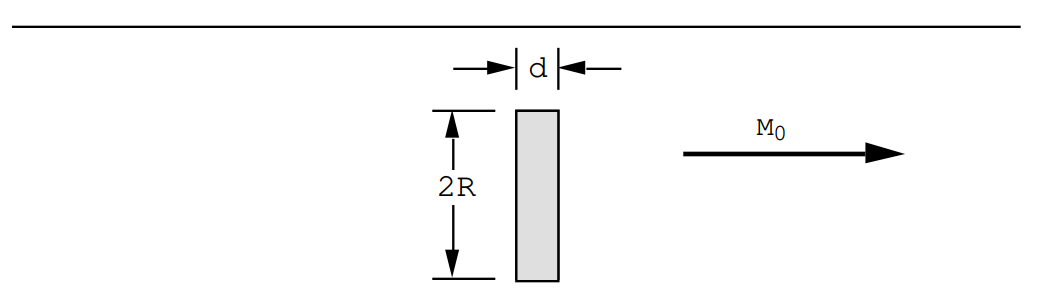This problem can be worked as the superposition of a uniformly magnetized, infinitely long rod plus a uniformly magnetized disc, but for the disc Mz= - M0. For the uniform rod Bz= µ0M0. Along the axis of the disc

$\text{B}_{\text{z}}(\text{z})=-\frac{\mu_{0} \text{M}_{0}}{2}\left(\frac{(\text{z}+\text{d} / 2)}{\sqrt{(\text{z}+\text{d} / 2)^{2}+\text{R}^{2}}}+\frac{(\text{d} / 2-\text{z})}{\sqrt{(\text{z}-\text{d} / 2)^{2}+\text{R}^{2}}}\right)\nonumber,$

and at z=0

$\text{B}_{\text{z}}(0)=-\frac{\mu_{0} \text{M}_{0}}{2} \frac{\text{d}}{\sqrt{(\text{d} / 2)^{2}+\text{R}^{2}}}\nonumber.$

The total field at the center of the disc will be

$\text{B}_{\text{z}}(0)=\mu_{0} \text{M}_{0}\left(1-\frac{\text{d}}{2 \sqrt{(\text{d} / 2)^{2}+\text{R}^{2}}}\right)\nonumber.$

In the limit (d/R)→0 the field at the center of the cavity is just B0= µ0M0.

Problem (4.13)

A uniformly magnetized ellipsoid possesses magnetization components

$$\begin{array}{l} \text{M}_{\text{X}}=2 \times 10^{5} \text { Amps/meter, } \\ \text{M}_{\text{Y}}=2 \times 10^{5} \text { Amps/meter } \\ \text{M}_{\text{Z}}=4 \times 10^{5} \text { Amps/meter } \end{array}$$

when referred to the principle axes of the ellipsoid. Demagnetizing coefficients for the ellipsoid are

$$\begin{array}{l} \text{N}_{\text{X}}=0.2, \\ \text{N}_{\text{Y}}=0.3 \end{array}.$$

(a) Calculate the components of H inside the ellipsoid.

(b) Calculate the components of B inside the ellipsoid.

(c) Calculate the angle between B and M.

The demagnetizing coefficients obey the sum rule

$$\text{N}_{\text{x}}+\text{N}_{\text{y}}+\text{N}_{\text{z}}=1$$.

For this problem

$$\begin{array}{l} \text{N}_{\text{x}}=0.20, \\ \text{N}_{\text{Y}}=0.30 ,\\ \text{N}_{\text{z}}=0.50. \end{array}$$

(a) $$\begin{array}{l} \text{H}_{\text{X}}=-\text{N}_{\text{X}} \quad \quad \text{M}_{\text{X}}=-0.40 \times 10^{5} \text { Amps/meter. } \\ \text{H}_{\text{Y}}=-\text{N}_{\text{Y}} \quad \quad \text{M}_{\text{Y}}=-0.60 \times 10^{5} \text { Amps/meter. } \\ \text{H}_{\text{Z}}=-\text{N}_{\text{Z}} \quad \quad \text{M}_{\text{Z}}=-2.00 \times 10^{5} \text { Amps/meter. } \end{array}$$

(b) $$\mathbf{B}=\boldsymbol{\mu}_{0}(\mathbf{H}+\mathbf{M})$$, therefore

$$\begin{array}{l} \text{B}_{\text{X}}=\mu_{0}\left(\text{H}_{\text{X}}+\text{M}_{\text{X}}\right)=0.201 \text { Teslas. } \\ \text{B}_{\text{Y}}=\mu_{0}\left(\text{H}_{\text{Y}}+\text{M}_{\text{Y}}\right)=0.176 \text { Teslas. } \\ \text{B}_{\text{z}}=\mu_{0}\left(\text{H}_{\text{Z}}+\text{M}_{\text{Z}}\right)=0.251 \text { Teslas. } \end{array}$$

(c) $$\mathbf{B} \cdot \mathbf{M}=|\mathbf{B}||\mathbf{M}| \quad \cos \theta$$;

$$|\mathbf{M}|=4.899 \times 10^{5} \text { Amps/meter }$$,

$$|\mathbf{B}|=0.367\ Teslas,$$

$$\cos \theta=\frac{M_{x} B_{x}+M_{y} B_{y}+M_{z} B_{z}}{|\mathbf{M}||\mathbf{B}|}=\frac{1.758}{(4.899)(.3667)}=0.9786$$.

So θ = 11.9°.

Problem (4.14)

A very large disc whose radius is infinite is magnetized along its normal as shown in the figure.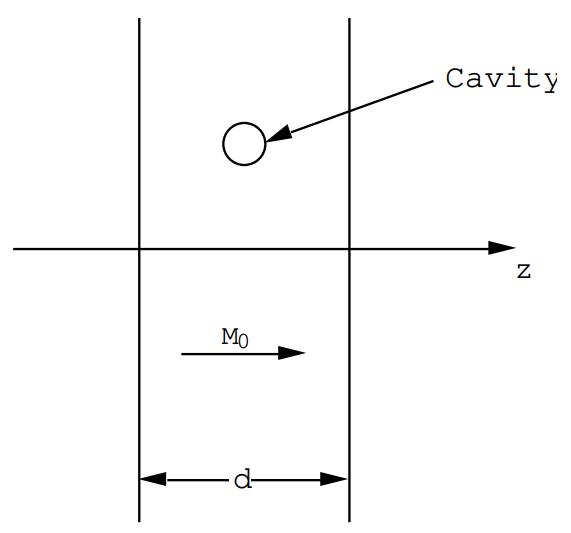(a) What is H in the disc?

(b) What is H outside the disc?

(c) What is B inside the slab?

(d) A spherical cavity is cut out of the material of the disc. Use the principle of superposition to calculate the magnetic field B in the cavity.

(a) The demagnetizing factor for the direction along the disc normal is Nz = 1. Therefore Hz = - Mo .

(b) Outside the disc the field is zero by analogy with the equivalent electrostatic problem i.e. two infinite charge sheets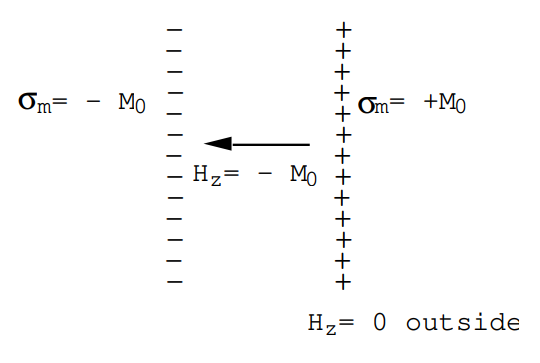(c) Bz = µo (Hz + Mz) ≡ 0.

(d) Inside a uniformly polarized sphere $$B_{z}=\frac{2}{3} \mu_{0} M_{0}$$. Therefore in the cavity one must have $$\text{B}_{\text{z}}=-\frac{2}{3} \mu_{\text{0}} \text{M}_{\text{0}}$$ so that the sum of the two fields gives zero when the sphere is put into the hole.

Problem (4.15)

A very long cylinder of magnetic material has a radius R. The axis of the cylinder lies along the z-axis. The magnetization depends upon the distance from the cylinder axis:

$\text{M}_{\text{z}}=\text{M}_{\text{0}}(\text{r} / \text{R}) \quad \text { Amps / meter. }\nonumber$

(a) Calculate the effective current density curl M both inside and outside the cylinder.

(b) Note that there is an effective surface current density on the surface of the cylinder due to the discontinuity in the tangential component of the magnetization. Calculate this surface current density, Js.

(c) Calculate the radial dependence of the magnetic field in the cylinder.

$\operatorname{curl} \mathbf{M}=\frac{1}{r}\left|\begin{array}{ccc} \hat{\mathbf{u}}_{r} & r \hat{\mathbf{u}}_{\theta} & \hat{\mathbf{u}}_{z} \\ \frac{\partial}{\partial r} & 0 & 0 \\ 0 & 0 & \text{M}_{z} \end{array}\right|=\left|\begin{array}{c} 0 \\ -\frac{\partial M_{z}}{\partial r} \\ 0 \end{array}\right|\nonumber,$

(There is no angular or z dependence).

(a) ∴ $$\text{J}_{\theta}=-\frac{\partial \text{M}_{\text{z}}}{\partial \text{r}}=-\text{M}_{\text{0}} / \text{R}$$ ie. independent of position.

(b) At the outer surface there is a discontinuity in the tangential component of M. Use Stokes' theorem to obtain the effective surface current density:

Jb = curl M

$\therefore \quad \int_{\text {surface }} \mathbf{J}_{b}\cdot \mathbf{d s}=\int_{\text {surface }} \operatorname{curl} \mathbf{M} \cdot \mathbf{d s}=\oint_{C} \mathbf{M} \cdot \text{d} \mathbf{L}\nonumber$

Apply this to the loop shown below:Current through the loop $$\int \mathbf{J}_{\text{b}} \cdot \mathbf{d s}=J_{\text{s}} \text{L}$$

Js is the effective surface current density.

$$\oint_{C} \mathbf{M} \cdot \text{d} \mathbf{L}=\text{M}_{\text{0}} \text{L}$$

$$\therefore \quad J_{S} L=M_{0} L$$

or Js = Mo Amps/m.

(c) Calculate the field along the axis of the cylinder. By symmetry there is only a z-component which is independent of z. The uniform effective current density, $$-\frac{\text{M}_{0}}{\text{R}}$$, can be treated like a nested solenoid problem in order to calculate the magnetic field along the cylinder axis.

The effective current sheet strength is $$\text{NI}=-\left(\frac{\text{M}_{\text{0}}}{\text{R}}\right) \text{dr} \quad \text{Amps} / \text{m}$$.

This produces the solenoid field contribution $$\text{dB}_{\text{z}}=-\mu_{\text{0}}\left(\frac{\text{M}_{\text{0}}}{\text{R}}\right) \text{dr}$$.

Integrate from r = 0 to r = R: Bz = - µoMo Tesla. However, this is just cancelled by the surface current sheet which produces Bz = µoMo Tesla.

∴ On the axis Bz ≡ 0.

Now use curl B = µo curl M

or $$\oint_{C} \mathbf{B} \cdot d \mathbf{L}=\mu_{0} \quad \int_{C} \mathbf{M} \cdot \mathbf{d} \mathbf{L}$$ and integrate around the loop shown in the figure:from which Bz = µoMz

∴ Bz(r) = µoMo(r/R)

and Hz ≡ 0 everywhere.

Problem (4.16)

A permanent magnet is formed in the shape of a dough-nut having an inner radius a meters and an outer radius of b meters (see the figure). The magnetization density has the components Mr=0, Mθ= M0, Mz=0 in cylindrical polar coordinates, where M0 is constant.(a) Calculate the field H everywhere.

(Answ: div M=0 everywhere, and there are no free currents. Therefore there are no sources for H and consequently H=0 everywhere.)

(b) Suppose that a gap d meters wide is opened in the ring as shown in the figure. Calculate the field B at the center of the gap.

(Answ: $$\text{B}_{0}=\mu_{0} \text{M}_{0}\left(1-\frac{\text{d}}{\sqrt{4(\text{b}-\text{a})^{2}+\text{d}^{2}}}\right) \text { Teslas. }$$)

A uniform magnetic charge density will appear on the faces of the cut due to the discontinuity in M. The surface charge density on the left hand face is +M0/m2; the surface charge density on the right hand face is -M0/m2. These charge distributions produce a field at the gap center given by

$\text{H}_{0}=\frac{\text{M}_{0} \text{d}}{4} \int_{0}^{\text{R}} 2 \text{rdr} \frac{1}{\left(r^{2}+(d / 2)^{2}\right)^{3 / 2}}\nonumber$

where R= (b-a)/2.

$\text{H}_{0}=\text{M}_{0} \quad\left(1-\frac{\text{d}}{\sqrt{(\text{b}-\text{a})^{2}+\text{d}^{2}}}\right)\nonumber,$

B0= µ0H0 directed along M0, ie along -x in the above figure. This problem can also be solved by treating the magnetized plug removed from the gap as a short solenoid: for a short solenoid of radius R= (b-a)/2 and of length d the field at its center is given by

$B_{x}=-\frac{\mu_{0} M_{0}}{2} \frac{d}{\sqrt{\left(R^{2}+(d / 2)^{2}\right)}}\nonumber.$

This field plus the gap field, $$B_{x}^{G}$$, must equal the field in the gapless ring, - µ0M0, by superposition. Therefore

$B_{x}^{G}=-\mu_{0} M_{0}\left(1-\frac{d / 2}{\sqrt{(d / 2)^{2}+R^{2}}}\right)=-\mu_{0} M_{0}\left(1-\frac{d}{\sqrt{d^{2}+(b-a)^{2}}}\right)\nonumber,$